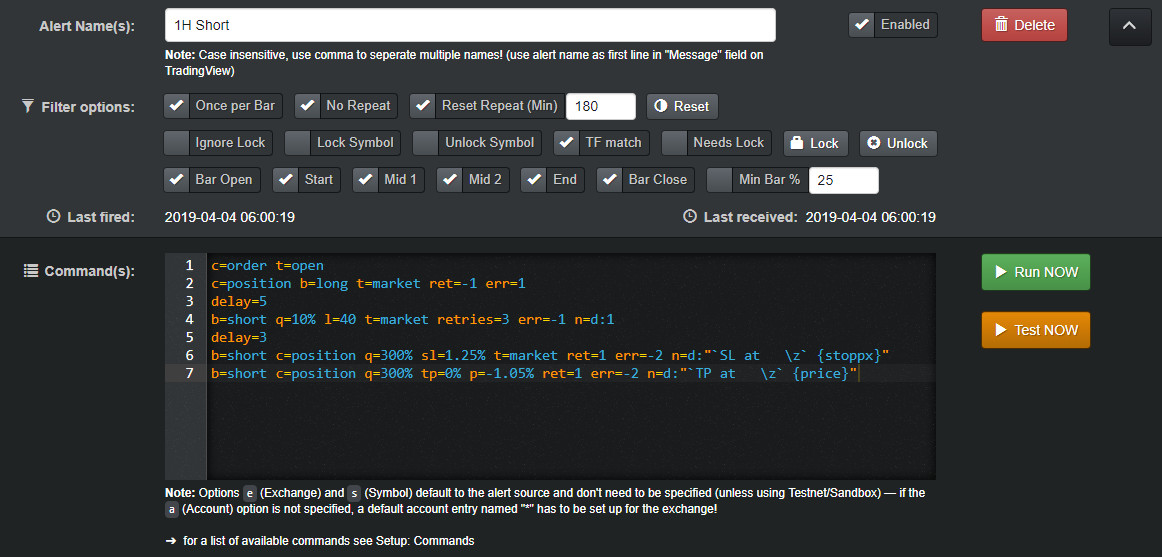#### Long Signal

``````### Deribit Long ###

# Optionally for multiple accounts uncomment the following line and fill in your accounts
#ap=acc1,acc2,acc3

# cancel all orders
c=order
# close shorts at market, if no position skip delay
c=position b=short t=market ret=-1 err=1
delay=5

# open long at market with some % of the balance
# note: since deribit does not have isolated margin
# the l= parameter will just multiply your buy in by the set amount
b=long q=YOUR_BUY_IN% l=YOUR_LEVERAGE t=market err=-1 retries=3
delay=3

# place a market stoploss order (expect to return an order)
b=long c=position q=100% sl=-YOUR_STOP_LOSS% t=market ret=1 err=-2 retries=10 id=SL

# place a limit sell (=long close) order at profit (reduce only & expect to return an order)
b=short q=100% p=YOUR_TAKE_PROFIT% r=1 ret=1 err=-2 retries=10 id=TP
``````

`q= l= sl= p= has to be adjusted to your strategy / personal needs!`#### Short Signal

``````### Deribit Short ###

# Optionally for multiple accounts uncomment the following line and fill in your accounts
#ap=acc1,acc2,acc3

# cancel all orders
c=order
# close longs at market, if no position skip delay
c=position b=long t=market ret=-1 err=1
delay=5

# open short at market with some % of the balance
# note: since deribit does not have isolated margin
# the l= parameter will just multiply your buy in by the set amount
b=short q=YOUR_BUY_IN% l=YOUR_LEVERAGE t=market err=-1 retries=3
delay=3

# place a market stoploss order (expect to return an order)
b=short c=position q=100% sl=YOUR_STOP_LOSS% t=market ret=1 err=-2 retries=10 id=SL

# place a limit buy (=short close) order at profit (reduce only & expect to return an order)
b=long q=100% p=-YOUR_TAKE_PROFIT% r=1 ret=1 err=-2 retries=10 id=TP
``````

`q= l= sl= p= has to be adjusted to your strategy / personal needs!`#### Attention

Always double check that you are using the right syntax/alert!